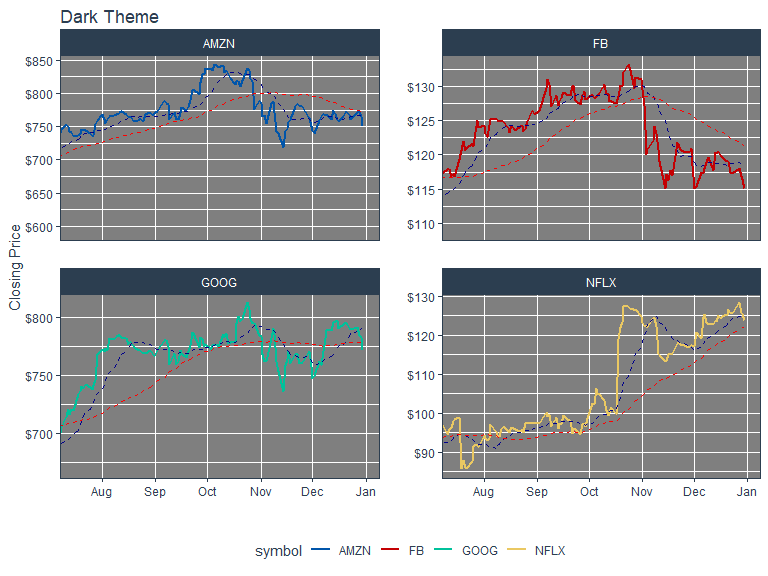# Charting with tidyquant

#### 2023-03-31

Charting financial data using ggplot2

# Overview

The tidyquant package includes charting tools to assist users in developing quick visualizations in ggplot2 using the grammar of graphics format and workflow. There are currently three primary geometry (geom) categories and one coordinate manipulation (coord) category within tidyquant:

• Chart Types: Two chart type visualizations are available using geom_barchart and geom_candlestick.
• Moving Averages: Seven moving average visualizations are available using geom_ma.
• Bollinger Bands: Bollinger bands can be visualized using geom_bbands. The BBand moving average can be one of the seven available in Moving Averages.
• Zooming in on Date Ranges: Two coord functions are available (coord_x_date and coord_x_datetime), which prevent data loss when zooming in on specific regions of a chart. This is important when using the moving average and Bollinger band geoms.

# Prerequisites

Load the tidyquant package to get started.

# Loads tidyquant, lubridate, xts, quantmod, TTR, and PerformanceAnalytics
library(tidyverse)
library(tidyquant)  

The following stock data will be used for the examples. Use tq_get to get the stock prices.

# Use FANG data set
data("FANG")

# Get AAPL and AMZN Stock Prices
AAPL <- tq_get("AAPL", get = "stock.prices", from = "2015-09-01", to = "2016-12-31")
AMZN <- tq_get("AMZN", get = "stock.prices", from = "2000-01-01", to = "2016-12-31")

The end date parameter will be used when setting date limits throughout the examples.

end <- as_date("2016-12-31")

end
##  "2016-12-31"

The AAPL_range_60 will be used to adjust the zoom on the plots.

aapl_range_60_tbl <- AAPL %>%
tail(60) %>%
summarise(
max_high = max(high),
min_low  = min(low)
)

aapl_range_60_tbl
## # A tibble: 1 x 2
##   max_high min_low
##      <dbl>   <dbl>
## 1     29.7    26.0

# Chart Types

Financial charts provide visual cues to open, high, low, and close prices. The following chart geoms are available:

## Line Chart

Before we visualize bar charts and candlestick charts using the tidyquant geoms, let’s visualize stock prices with a simple line chart to get a sense of the “grammar of graphics” workflow. This is done using the geom_line from the ggplot2 package. The workflow begins with the stock data, and uses the pipe operator (%>%) to send to the ggplot() function.

The primary features controlling the chart are the aesthetic arguments: these are used to add data to the chart by way of the aes() function. When added inside the ggplot() function, the aesthetic arguments are available to all underlying layers. Alternatively, the aesthetic arguments can be applied to each geom individually, but typically this is minimized in practice because it duplicates code. We set aesthetic arguments, x = date and y = close, to chart the closing price versus date. The geom_line() function inherits the aesthetic arguments from the ggplot() function and produces a line on the chart. Labels are added separately using the labs() function. Thus, the chart is built from the ground up by starting with data and progressively adding geoms, labels, coordinates / scales and other attributes to create a the final chart. This is enables maximum flexibility wherein the analyst can create very complex charts using the “grammar of graphics”.

AAPL %>%
ggplot(aes(x = date, y = close)) +
geom_line() +
labs(title = "AAPL Line Chart", y = "Closing Price", x = "") +
theme_tq()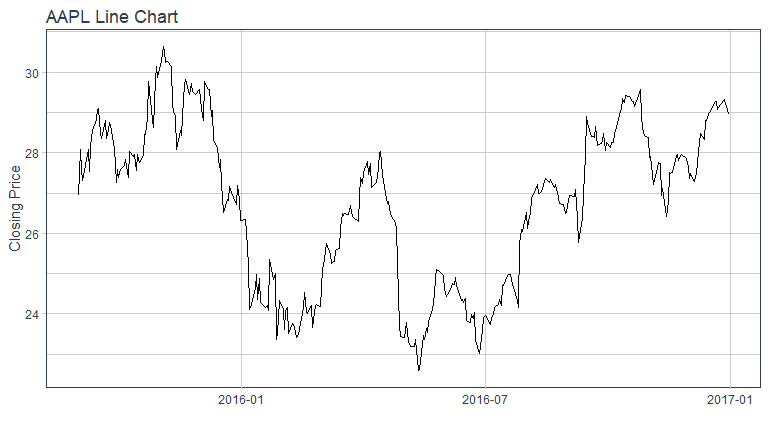## Bar Chart

Visualizing the bar chart is as simple as replacing geom_line with geom_barchart in the ggplot workflow. Because the bar chart uses open, high, low, and close prices in the visualization, we need to specify these as part of the aesthetic arguments, aes(). We can do so internal to the geom or in the ggplot() function.

AAPL %>%
ggplot(aes(x = date, y = close)) +
geom_barchart(aes(open = open, high = high, low = low, close = close)) +
labs(title = "AAPL Bar Chart", y = "Closing Price", x = "") +
theme_tq()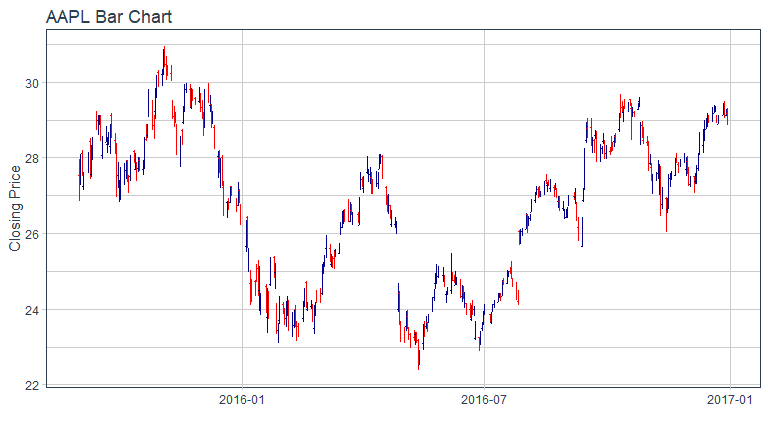We zoom into specific sections using coord_x_date, which has xlim and ylim arguments specified as c(start, end) to focus on a specific region of the chart. For xlim, we’ll use lubridate to convert a character date to date class, and then subtract six weeks using the weeks() function. For ylim we zoom in on prices in the range from 100 to 120.

AAPL %>%
ggplot(aes(x = date, y = close)) +
geom_barchart(aes(open = open, high = high, low = low, close = close)) +
labs(title = "AAPL Bar Chart",
subtitle = "Zoomed in using coord_x_date",
y = "Closing Price", x = "") +
coord_x_date(xlim = c(end - weeks(6), end),
ylim = c(aapl_range_60_tbl$min_low, aapl_range_60_tbl$max_high)) +
theme_tq()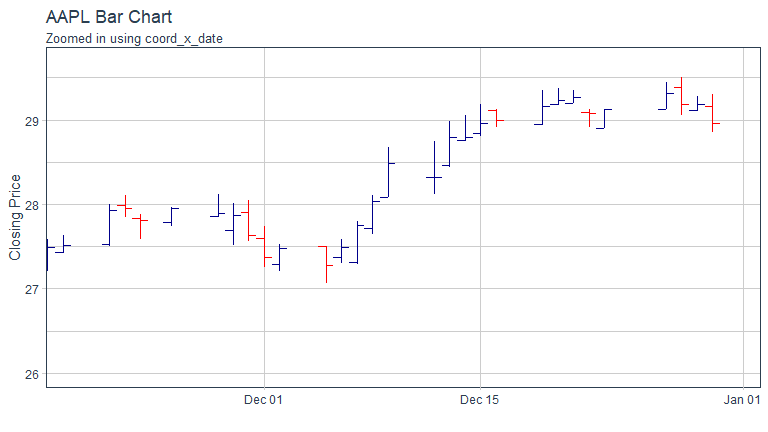The colors can be modified using colour_up and colour_down arguments, and parameters such as size can be used to control the appearance.

AAPL %>%
ggplot(aes(x = date, y = close)) +
geom_barchart(aes(open = open, high = high, low = low, close = close),
colour_up = "darkgreen", colour_down = "darkred", size = 1) +
labs(title = "AAPL Bar Chart",
subtitle = "Zoomed in, Experimenting with Formatting",
y = "Closing Price", x = "") +
coord_x_date(xlim = c(end - weeks(6), end),
c(aapl_range_60_tbl$min_low, aapl_range_60_tbl$max_high)) +
theme_tq()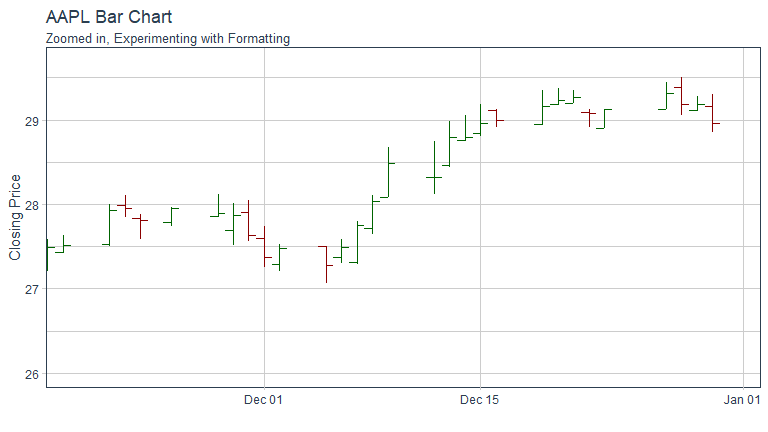## Candlestick Chart

Creating a candlestick chart is very similar to the process with the bar chart. Using geom_candlestick, we can insert into the ggplot workflow.

AAPL %>%
ggplot(aes(x = date, y = close)) +
geom_candlestick(aes(open = open, high = high, low = low, close = close)) +
labs(title = "AAPL Candlestick Chart", y = "Closing Price", x = "") +
theme_tq()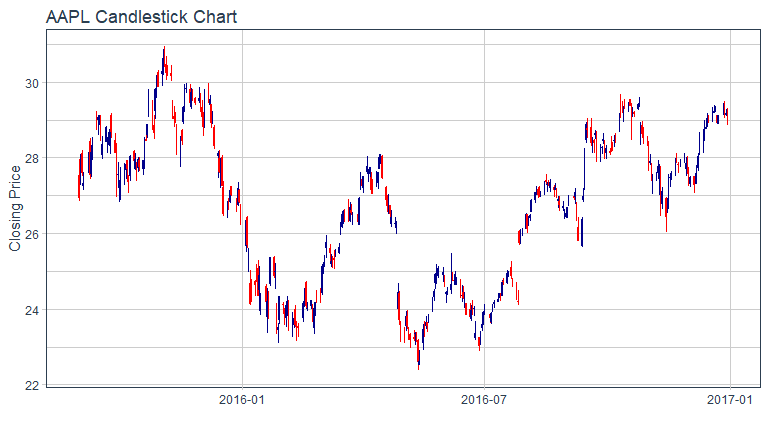We zoom into specific sections using coord_x_date.

AAPL %>%
ggplot(aes(x = date, y = close)) +
geom_candlestick(aes(open = open, high = high, low = low, close = close)) +
labs(title = "AAPL Candlestick Chart",
subtitle = "Zoomed in using coord_x_date",
y = "Closing Price", x = "") +
coord_x_date(xlim = c(end - weeks(6), end),
c(aapl_range_60_tbl$min_low, aapl_range_60_tbl$max_high)) +
theme_tq()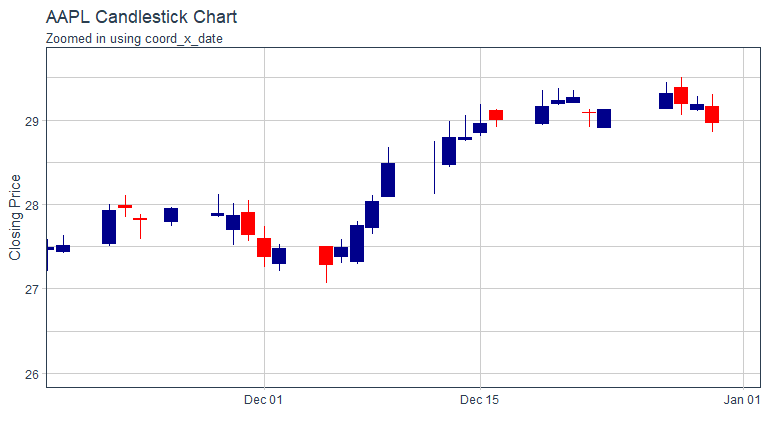The colors can be modified using colour_up and colour_down, which control the line color, and fill_up and fill_down, which control the rectangle fills.

AAPL %>%
ggplot(aes(x = date, y = close)) +
geom_candlestick(aes(open = open, high = high, low = low, close = close),
colour_up = "darkgreen", colour_down = "darkred",
fill_up  = "darkgreen", fill_down  = "darkred") +
labs(title = "AAPL Candlestick Chart",
subtitle = "Zoomed in, Experimenting with Formatting",
y = "Closing Price", x = "") +
coord_x_date(xlim = c(end - weeks(6), end),
c(aapl_range_60_tbl$min_low, aapl_range_60_tbl$max_high)) +
theme_tq()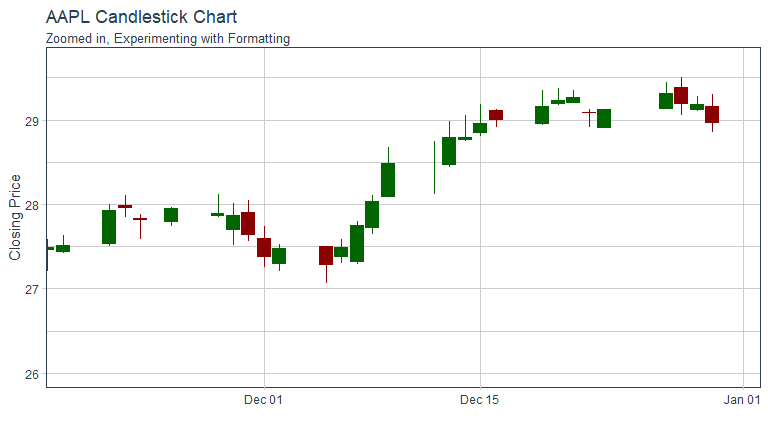## Charting Multiple Securities

We can use facet_wrap to visualize multiple stocks at the same time. By adding a group aesthetic in the main ggplot() function and combining with a facet_wrap() function at the end of the ggplot workflow, all four “FANG” stocks can be viewed simultaneously. You may notice an odd filter() call before the call to ggplot(). I’ll discuss this next.

start <- end - weeks(6)
FANG %>%
filter(date >= start - days(2 * 15)) %>%
ggplot(aes(x = date, y = close, group = symbol)) +
geom_candlestick(aes(open = open, high = high, low = low, close = close)) +
labs(title = "FANG Candlestick Chart",
subtitle = "Experimenting with Mulitple Stocks",
y = "Closing Price", x = "") +
coord_x_date(xlim = c(start, end)) +
facet_wrap(~ symbol, ncol = 2, scale = "free_y") +
theme_tq()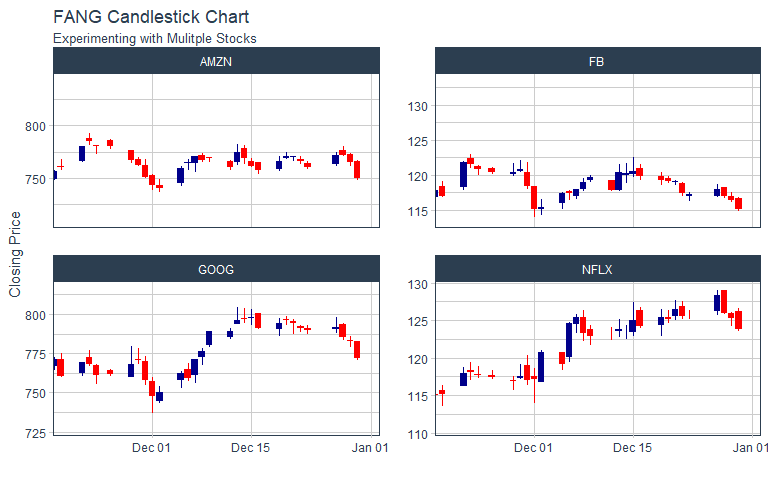A note about out-of-bounds data (or “clipping”), which is particularly important with faceting and charting moving averages:

The coord_x_date coordinate function is designed to zoom into specific sections of a chart without “clipping” data that is outside of the view. This is in contrast to scale_x_date, which removes out-of-bounds data from the charting. Under normal circumstances clipping is not a big deal (and is actually helpful for scaling the y-axis), but with financial applications users want to chart rolling/moving averages, lags, etc that depend on data outside of the view port. Because of this need for out-of-bounds data, there is a trade-off when charting: Too much out-of-bounds data distorts the scale of the y-axis, and too little and we cannot get a moving average. The optimal method is to include “just enough” out-of-bounds data to get the chart we want. This is why below the FANG data is filtered by date from double the number of moving-average days (2 * n) previous to the start date. This yields a nice y-axis scale and still allows us to create a moving average line using geom_ma.

start <- end - weeks(6)
FANG %>%
filter(date >= start - days(2 * 15)) %>%
ggplot(aes(x = date, y = close, group = symbol)) +
geom_candlestick(aes(open = open, high = high, low = low, close = close)) +
geom_ma(ma_fun = SMA, n = 15, color = "darkblue", size = 1) +
labs(title = "FANG Candlestick Chart",
subtitle = "Experimenting with Mulitple Stocks",
y = "Closing Price", x = "") +
coord_x_date(xlim = c(start, end)) +
facet_wrap(~ symbol, ncol = 2, scale = "free_y") +
theme_tq()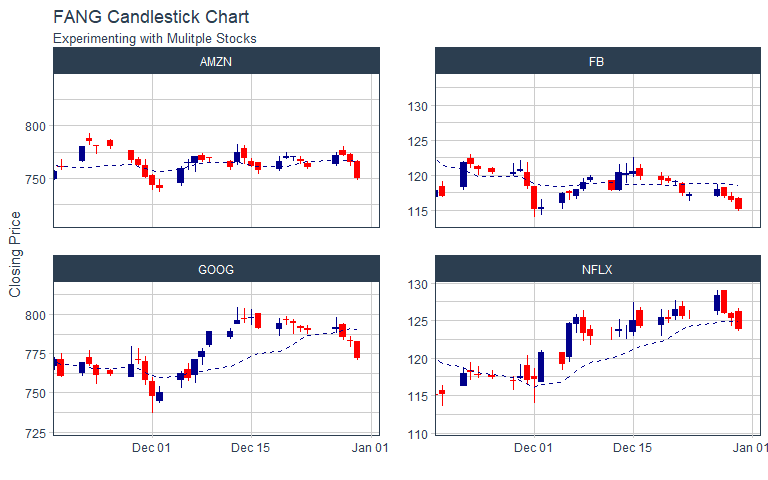# ggplot2 Functionality

Base ggplot2 has a ton of functionality that can be useful for analyzing financial data. We’ll go through some brief examples using Amazon (AMZN).

## Example 1: Log Scale with scale_y_log10

ggplot2 has the scale_y_log10() function to scale the y-axis on a logarithmic scale. This is extremely helpful as it tends to expose linear trends that can be analyzed.

Continuous Scale:

AMZN %>%
ggplot(aes(x = date, y = adjusted)) +
geom_line(color = palette_light()[]) +
scale_y_continuous() +
labs(title = "AMZN Line Chart",
subtitle = "Continuous Scale",
y = "Closing Price", x = "") +
theme_tq()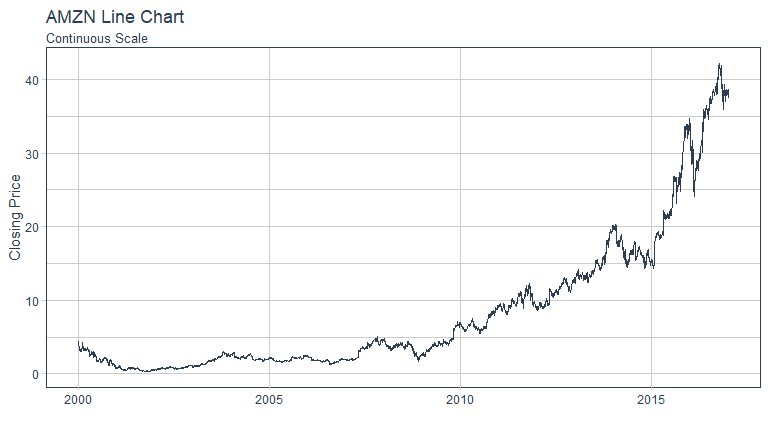Log Scale:

AMZN %>%
ggplot(aes(x = date, y = adjusted)) +
geom_line(color = palette_light()[]) +
scale_y_log10() +
labs(title = "AMZN Line Chart",
subtitle = "Log Scale",
y = "Closing Price", x = "") +
theme_tq()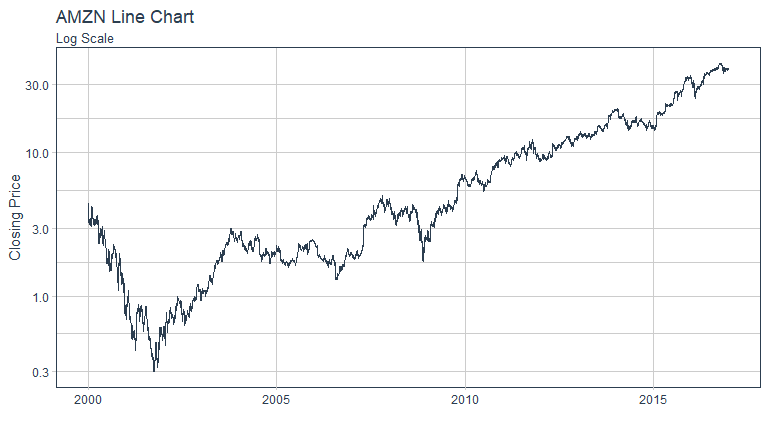## Example 2: Regression trendlines with geom_smooth

We can apply a trend line quickly adding the geom_smooth() function to our workflow. The function has several prediction methods including linear ("lm") and loess ("loess") to name a few.

Linear:

AMZN %>%
ggplot(aes(x = date, y = adjusted)) +
geom_line(color = palette_light()[]) +
scale_y_log10() +
geom_smooth(method = "lm") +
labs(title = "AMZN Line Chart",
subtitle = "Log Scale, Applying Linear Trendline",
y = "Adjusted Closing Price", x = "") +
theme_tq()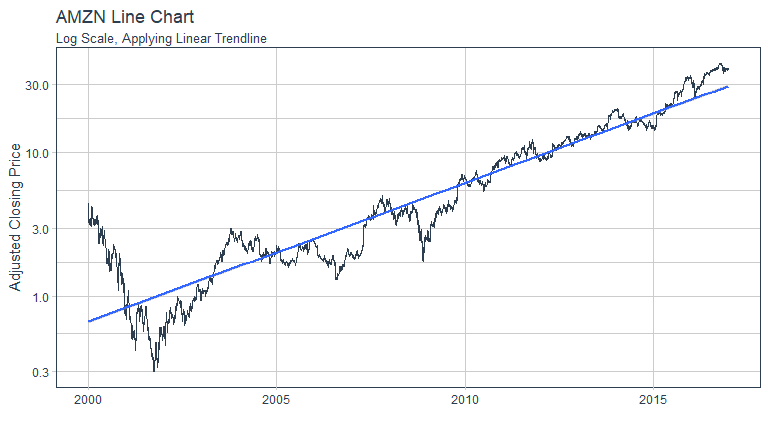## Example 3: Charting volume with geom_segment

We can use the geom_segment() function to chart daily volume, which uses xy points for the beginning and end of the line. Using the aesthetic color argument, we color based on the value of volume to make these data stick out.

AMZN %>%
ggplot(aes(x = date, y = volume)) +
geom_segment(aes(xend = date, yend = 0, color = volume)) +
geom_smooth(method = "loess", se = FALSE) +
labs(title = "AMZN Volume Chart",
subtitle = "Charting Daily Volume",
y = "Volume", x = "") +
theme_tq() +
theme(legend.position = "none")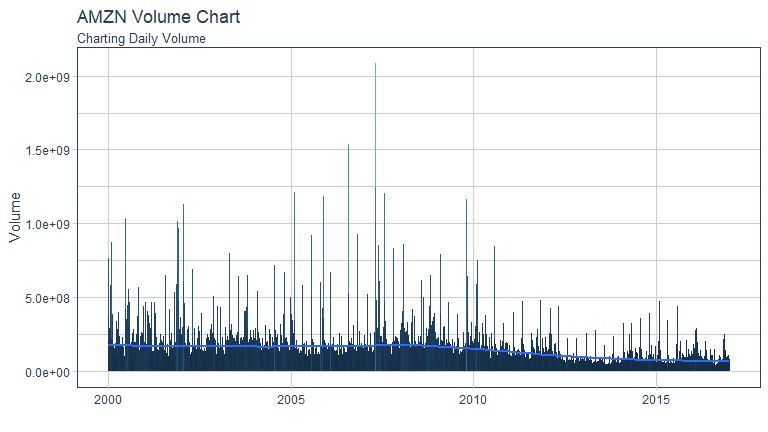And, we can zoom in on a specific region. Using scale_color_gradient we can quickly visualize the high and low points, and using geom_smooth we can see the trend.

start <- end - weeks(24)
AMZN %>%
filter(date >= start - days(50)) %>%
ggplot(aes(x = date, y = volume)) +
geom_segment(aes(xend = date, yend = 0, color = volume)) +
geom_smooth(method = "loess", se = FALSE) +
labs(title = "AMZN Bar Chart",
subtitle = "Charting Daily Volume, Zooming In",
y = "Volume", x = "") +
coord_x_date(xlim = c(start, end)) +
scale_color_gradient(low = "red", high = "darkblue") +
theme_tq() +
theme(legend.position = "none")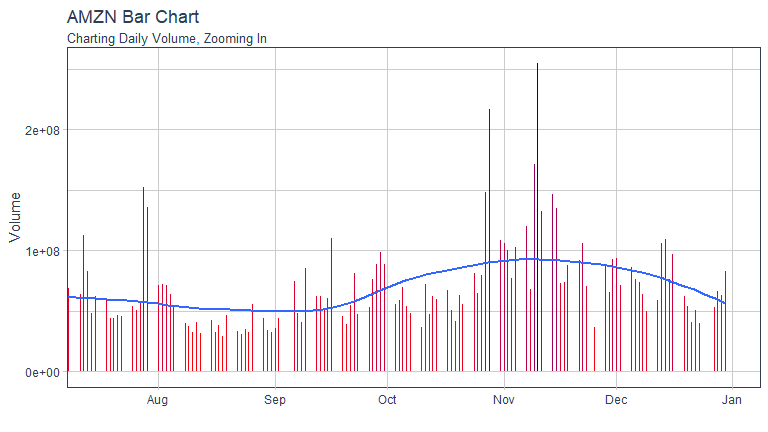# Themes

The tidyquant package comes with three themes to help quickly customize financial charts:

• Light: theme_tq() + scale_color_tq() + scale_fill_tq()
• Dark: theme_tq_dark() + scale_color_tq(theme = "dark") + scale_fill_tq(theme = "dark")
• Green: theme_tq_green() + scale_color_tq(theme = "green") + scale_fill_tq(theme = "green")

## Dark

n_mavg <- 50 # Number of periods (days) for moving average
FANG %>%
filter(date >= start - days(2 * n_mavg)) %>%
ggplot(aes(x = date, y = close, color = symbol)) +
geom_line(size = 1) +
geom_ma(n = 15, color = "darkblue", size = 1) +
geom_ma(n = n_mavg, color = "red", size = 1) +
labs(title = "Dark Theme",
x = "", y = "Closing Price") +
coord_x_date(xlim = c(start, end)) +
facet_wrap(~ symbol, scales = "free_y") +
theme_tq_dark() +
scale_color_tq(theme = "dark") +
scale_y_continuous(labels = scales::dollar)